Courses

# Design of Friction Drives - MCQ Test 2

## 30 Questions MCQ Test RRB JE for Mechanical Engineering | Design of Friction Drives - MCQ Test 2

Description
This mock test of Design of Friction Drives - MCQ Test 2 for Mechanical Engineering helps you for every Mechanical Engineering entrance exam. This contains 30 Multiple Choice Questions for Mechanical Engineering Design of Friction Drives - MCQ Test 2 (mcq) to study with solutions a complete question bank. The solved questions answers in this Design of Friction Drives - MCQ Test 2 quiz give you a good mix of easy questions and tough questions. Mechanical Engineering students definitely take this Design of Friction Drives - MCQ Test 2 exercise for a better result in the exam. You can find other Design of Friction Drives - MCQ Test 2 extra questions, long questions & short questions for Mechanical Engineering on EduRev as well by searching above.
QUESTION: 1

### A clutch has outer and inner diameters 100 mm and 40 mm respectively. Assuming a uniform pressure of 2 MPa and coefficient of friction of liner material 0.4, the torque carrying capacity of the clutch is

Solution: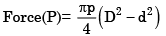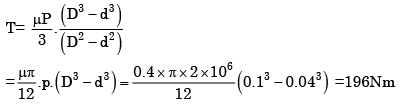QUESTION: 2

Solution:
QUESTION: 3

### With regard to belt drives with given pulley diameters, centre distance and coefficient of friction between the pulley and the belt materials, which of the statement below are FALSE?

Solution:
QUESTION: 4

What is the efficiency of a self-locking power screw?

Solution:

We know that the frictional torque for square thread at mean radius while raising load is given by tan(ø −α )

Where: (W = load;  Ro = Mean Radius; φ = Angle of friction;  α = Helix angle)    For self locking, angle of friction should be greater than helix angle of screw So that WRo tan(ø −α ) will become positive. i.e. we have to give torque to lowering the load.

QUESTION: 5

Which one of the following is the value of helix angle for maximum efficiency of a square threaded screw? [ø = tan-1μ ]

Solution:
QUESTION: 6

Consider the following statements in respect of flexible couplings:

1. The flanges of flexible coupling are usually made of grey cast iron FG200.

2. In the analysis of flexible coupling, it is assumed that the power is transmitted by the shear resistance of the pins.

3. Rubber bushes with brass lining are provided to absorb misalignment between the two shafts.

Which of the statements given above are correct?

Solution:

Since the pin is subjected to bending and shear stresses, therefore the design must be checked either for the maximum principal stress or maximum shear stress theory.

QUESTION: 7

Which one of the following is not a friction clutch?

Solution:
QUESTION: 8

Which one of the following pairs of parameters and effects is not correctly matched?

Solution:
QUESTION: 9

Assertion (A): In case of friction clutches, uniform wear theory should be considered for power transmission calculation rather than the uniform pressure theory.

Reason (R): The uniform pressure theory gives a higher friction torque than the uniform wear theory.

Solution:

Uniform pressure theory is applicable only when the clutches are new i.e., the assumption involved is that axial force W is uniformly distributed. Moreover torque transmitted in uniform pressure is more hence for safety in design uniform wear theory is used.

QUESTION: 10

In a flat collar pivot bearing, the moment due to friction is proportional to (r1 and r2 are the outer and inner radii respectively)

Solution:
QUESTION: 11

In a multiple disc clutch if n1 and n2 are the number of discs on the driving and driven shafts, respectively, the number of pairs of contact surfaces will be

Solution:
QUESTION: 12

Which one of the following is the correct expression for the torque transmitted by a conical clutch of outer radius R, Inner radius r and semicone angle α assuming uniform pressure? (Where W = total axial load and μ = coefficient of friction)

Solution:
QUESTION: 13

Consider the following statements regarding a centrifugal clutch: It need not be unloaded before engagement.

1. It enables the prime mover to start up under no-load conditions.

2. It picks up the load gradually with the increase in speed

3. It will not slip to the point of destruction

4. It is very useful when the power unit has a low starting torque

Which of these are the advantages of centrifugal clutch?

Solution:
QUESTION: 14

The creep in a belt drive is due to the

Solution:

• When the belt passes from the slack side to the tight side, a certain portion of the belt extends and it contracts again when the belt passes from the tight side to the slack side. Due to these changes of length, there is a relative motion between the belt and the pulley surfaces. This relative motion is termed as creep. The total effect of creep is to reduce slightly the speed of the driven pulley or follower.

• Here english meaning of ‘creep’ is ‘very slow motion’ and not ‘When a part is subjected to a constant stress at high temperature for a long period of time, it will undergo a slow and permanent deformation called creep.’

• Therefore the belt creep is very slow motion between the belt and the pulley surfaces due to unequal tension on tight and slack sides of the belt.  • Don’t confuse with material of the belt because the belt creep depends on both the materials of the pulley and the materials of the belt.

QUESTION: 15

In flat belt drive, if the slip between the driver and the belt is 1%, that between belt and follower is 3% and driver and follower pulley diameters are equal, then the velocity ratio of the drive will be

Solution:
QUESTION: 16

The length of the belt in the case of a cross-belt drive is given in terms of centre distance between pulleys (C), diameters of the pulleys D and d as

Solution:
QUESTION: 17

Assertion (A ): In a short centre open-belt drive, an idler pulley is used to maintain the belt tension and to increase the angle of contact on the smaller pulley.

Reason (R): An idler pulley is free to rotate on its axis and is put on the slack side of the belt.

Solution:

Both A and R are true, and R provides correct explanation for A.

QUESTION: 18

In the case of a vertical belt pulley drive with Tc as centrifugal tension and To as the initial tension, the belt would tend to hang clear of the tower pulley when

Solution:
QUESTION: 19

In a flat belt drive the belt can be subjected to a maximum tension T and centrifugal tension Tc .What is the condition for transmission of maximum power?

Solution:

Condition for maximum power transmission:-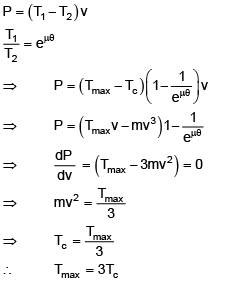QUESTION: 20

Which one of the following statements with regard to belt drives is NOT correct?

Solution:
QUESTION: 21

Assertion ( A): For similar materials having the same maximum permissible tension V-belt transmits more power than flat belt with same velocity ratio and centre distance.

Reason (R): As tw o sides of V-belt are in contact with side faces of pulley groove, larger contact area gives greater effective frictional force.

Solution:
QUESTION: 22

Consider the following:

V-belts are specified by their

1. Nominal inside length in mm

2. Nominal pitch length

3. Belt cross section symbol

4. weight/unit length of the belt

Which of the above are correct?

Solution: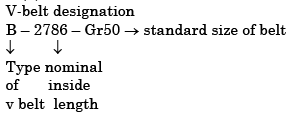QUESTION: 23

Which one of the following drives is used for a constant velocity ratio, positive drive with large centre distance between the driver and driven shafts?

Solution:
QUESTION: 24

Sources of power loss in a chain drive are given below:

1. Friction between chain and sprocket teeth.

2. Overcoming the chain stiffness.

3. Overcoming the friction in shaft bearing.

4. Frictional resistance to the motion of the chain in air or lubricant.

The correct sequence of descending order of power loss due to these sources is

Solution:

Power loss in descending order takes place as 1, 2 3 and 4.

QUESTION: 25

In a 6 × 20 wire rope, No.6 indicates the

Solution:

6×20 wire rope: 6 indicates number of strands in the wire rope and 20 indicates no of wire in a strand.

QUESTION: 26

Given that W = weight of load handled, Wr = weight of rope and f = acceleration, the additional load in ropes of a hoist during starting is given by

Solution:
QUESTION: 27

Power screws are used to produce uniform, slow and powerful motion such as required in presses, jacks and other machinery. 'V' threads are usually not used for this application due to low efficiency. This is because:

Solution: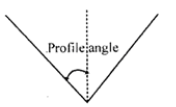Square thread most efficient. Profile angle is zero which causes excessive bursting force.

QUESTION: 28

The following parameters are to be calculated while designing screw jack.

1. Core diameter of screw

2. Torque required to rotate the screw

3. Principal stresses

4. Height of the nut

The correct sequence of the calculation of these parameters is

Solution:
QUESTION: 29

The diameter of tommy bar for a screw jack is designed for

Solution:
QUESTION: 30

The load cup of a screw jack is made separate from the head of the spindle to

Solution: